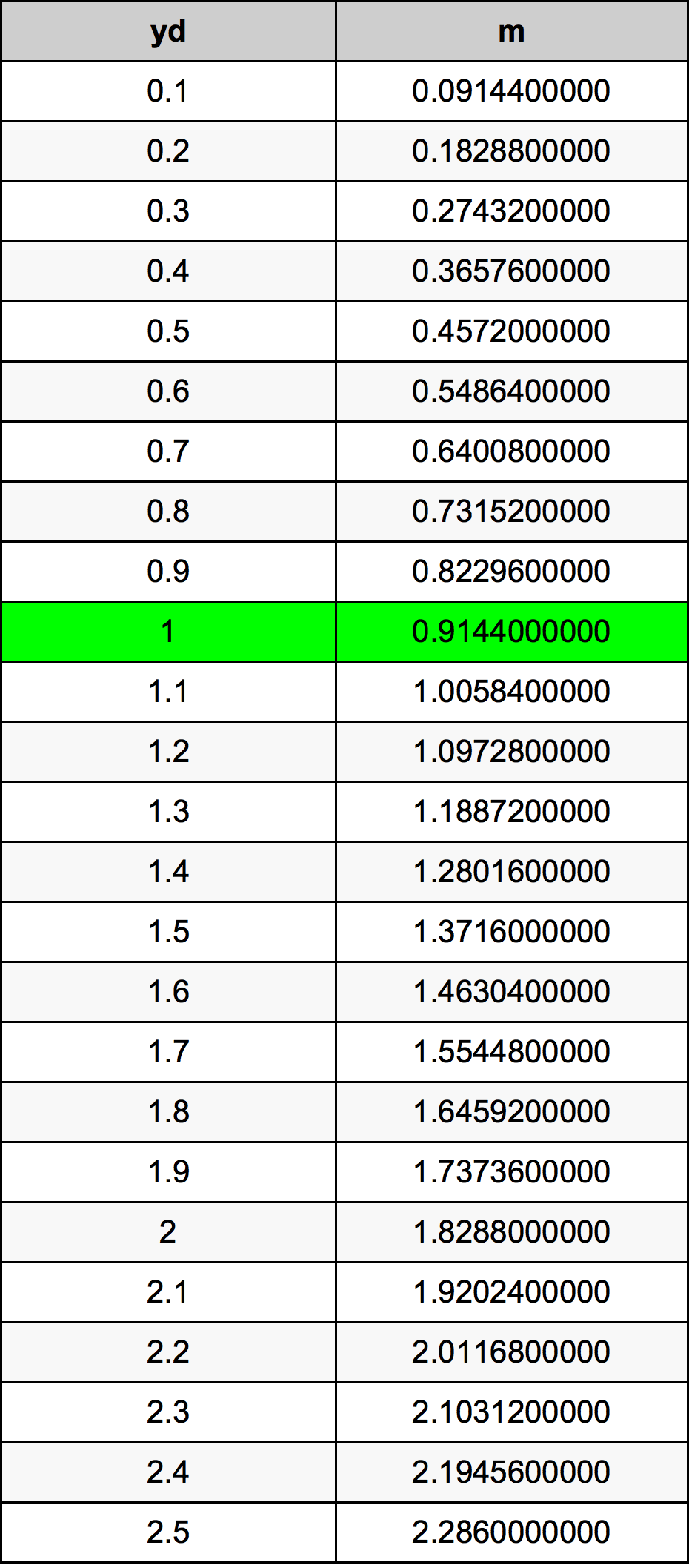Yards To Meters

# 1 yd to m1 Yard to Meters

yd
=
m

## How to convert 1 yard to meters?

 1 yd * 0.9144 m = 0.9144 m 1 yd
A common question is How many yard in 1 meter? And the answer is 1.0936132983 yd in 1 m. Likewise the question how many meter in 1 yard has the answer of 0.9144 m in 1 yd.

## How much are 1 yards in meters?

1 yards equal 0.9144 meters (1yd = 0.9144m). Converting 1 yd to m is easy. Simply use our calculator above, or apply the formula to change the length 1 yd to m.

## Convert 1 yd to common lengths

UnitLength
Nanometer914400000.0 nm
Micrometer914400.0 µm
Millimeter914.4 mm
Centimeter91.44 cm
Inch36.0 in
Foot3.0 ft
Yard1.0 yd
Meter0.9144 m
Kilometer0.0009144 km
Mile0.0005681818 mi
Nautical mile0.0004937365 nmi

## What is 1 yards in m?

To convert 1 yd to m multiply the length in yards by 0.9144. The 1 yd in m formula is [m] = 1 * 0.9144. Thus, for 1 yards in meter we get 0.9144 m.

## 1 Yard Conversion Table## Alternative spelling

1 Yards to Meter, 1 Yards in Meter, 1 Yard to Meters, 1 Yard in Meters, 1 Yards to m, 1 Yards in m, 1 Yards to Meters, 1 Yards in Meters, 1 yd to Meters, 1 yd in Meters, 1 Yard to m, 1 Yard in m, 1 Yard to Meter, 1 Yard in Meter